# AP Calculus AB Practice Test 45

### Test Information8 questions24 minutes

Calculator Allowed

1. If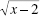is replaced by u, then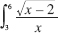is equivalent to

2. The line tangent to the graph of function f at the point (8,1) intersects the y-axis at y = 3. Find f (8).

3. How many points of inflection does the function f have on the interval 0 ≤ x ≤ 6 if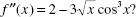4.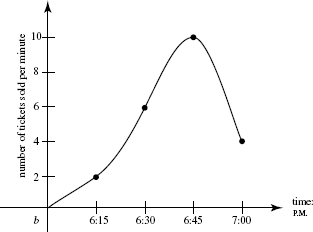The graph shows the rate at which tickets were sold at a movie theater during thelast hour before showtime. Using the right-rectangle method, estimate the size ofthe audience.

5. At what point of intersection of f (x) = 4sin x and g(x) = ln (x2) do their derivatives have the same sign?

6. Which statement is true?

7.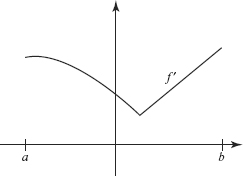The graph of f is shown above. Which statements about f must be true for a < x < b ?

I. f is increasing.

II. f is continuous.

III. f is differentiable.

8. After a bomb explodes, pieces can be found scattered around the center of the blast.The density of bomb fragments lying x meters from ground zero is given by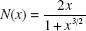fragments per square meter. How many fragments will be found within 20 meters ofthe point where the bomb exploded?